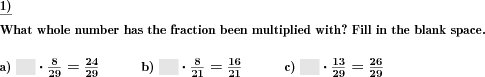Custom math worksheets at your fingertipsDetails for problem "Fractions multiplication by whole numbers fill blanks"

Quickname: 2501

Elementary School, Primary School, Junior High School, Middle School, High School.

Summary

What whole number has be fraction been multiplied with?

ExampleDescription

A product term with a fraction and a whole number is given, as is the result. The whole number has been replaced by a blank space. The correct number has to be filled in.

The number range for the numerator, denominator and whole number can be specified.

Improper fractions can be allowed, disallowed or enforced. In case they may occur, it can be chosen whether they will be output as improper fractions or mixed numbers.

Download free printable worksheets for this math problem here. The worksheet contains the problems only, the solution sheet includes the answers. Just click on the respective link.

•Worksheet 1Solution sheet with answers
•Worksheet 2Solution sheet with answers
•Worksheet 3Solution sheet with answers

If you can not see the solution sheets for download, they may be filtered out by an ad blocker that you may have installed. If this is the case, please allow ads for this page and reload the page. The solution sheets will then reappear.

• Do these sample worksheets do not really fit?
• Do you need more math worksheets, with a different level of difficulty?
• Would you like to combine different problems on a worksheet and adjust them to your needs?
• As a teacher, you can put together your own worksheets using the automatically generated math problems provided.
With a free initial credit, you can start creating your own math worksheets in a few minutes.

You can try it for free! Register here, to create custom worksheets now!

Customization options for this problem

Parameter
Possible values
Number of problems
1, 2, 3, 4, 5, 6, 7, 8, 9, 10
Number range
10, 20, 30, 40, 50, 100, 200, 500, 1000, 10000, 100000
Improper fractions
No, Yes, as a fraction, Yes, as a mixed number

Similar problems

Remark
Description
As a plain whole number times a fraction multiplication problem
A fraction has to multiplied by a whole number.Deutsche Version dieser Aufgabe
These informational pages with samples describe math problems that can be combined on custom math worksheets with solutions for home and K-12 school use.
Deutsche Seiten
×